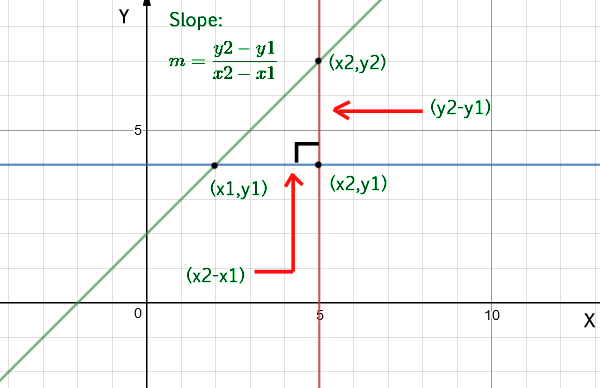GeeksforGeeks App
Open AppBrowser
Continue

# Program to find slope of a line

Given two coordinates, find the slope of a straight line.

Examples:

```Input  : x1 = 4, y1 = 2,
x2 = 2, y2 = 5
Output : Slope is -1.5```

Approach: To calculate the slope of a line you need only two points from that line, (x1, y1) and (x2, y2). The equation used to calculate the slope from two points is:Below is the implementation of the above approach:

## C++

 `// C++ program for slope of line``#include ``using` `namespace` `std;`` ` `// function to find the slope of a straight line``float` `slope(``float` `x1, ``float` `y1, ``float` `x2, ``float` `y2)``{``    ``if` `(x2 - x1 != 0)``        ``return` `(y2 - y1) / (x2 - x1);``    ``return` `INT_MAX;``}`` ` `// driver code to check the above function``int` `main()``{``    ``float` `x1 = 4, y1 = 2;``    ``float` `x2 = 2, y2 = 5;``    ``cout << ``"Slope is: "` `<< slope(x1, y1, x2, y2);``    ``return` `0;``}`

## Java

 `// Java program for slope of line``import` `java.io.*;`` ` `class` `GFG {``    ``static` `float` `slope(``float` `x1, ``float` `y1, ``float` `x2,``                       ``float` `y2)``    ``{``        ``if` `(x2 - x1 != ``0``)``            ``return` `(y2 - y1) / (x2 - x1);``        ``return` `Integer.MAX_VALUE;``    ``}``    ``public` `static` `void` `main(String[] args)``    ``{``        ``float` `x1 = ``4``, y1 = ``2``;``        ``float` `x2 = ``2``, y2 = ``5``;``        ``System.out.println(``"Slope is: "``                           ``+ slope(x1, y1, x2, y2));``    ``}``}`

## Python

 `# Python program for slope of line``def` `slope(x1, y1, x2, y2):``    ``if``(x2 ``-` `x1 !``=` `0``):``      ``return` `(``float``)(y2``-``y1)``/``(x2``-``x1)``    ``return` `sys.maxint`` ` ` ` `# driver code``x1 ``=` `4``y1 ``=` `2``x2 ``=` `2``y2 ``=` `5``print` `"Slope is:"``, slope(x1, y1, x2, y2)`

## C#

 `using` `System;`` ` `public` `static` `class` `GFG {``    ``// C# program for slope of line`` ` `    ``// function to find the slope of a straight line``    ``public` `static` `float` `slope(``float` `x1, ``float` `y1, ``float` `x2,``                              ``float` `y2)``    ``{``        ``if` `(x2 - x1 != 0F) {``            ``return` `(y2 - y1) / (x2 - x1);``        ``}``        ``return` `int``.MaxValue;``    ``}`` ` `    ``// driver code to check the above function``    ``internal` `static` `void` `Main()``    ``{``        ``float` `x1 = 4F;``        ``float` `y1 = 2F;``        ``float` `x2 = 2F;``        ``float` `y2 = 5F;``        ``Console.Write(``"Slope is: "``);``        ``Console.Write(slope(x1, y1, x2, y2));``    ``}``}`` ` `// The code is contributed by Aarti_Rathi`

## PHP

 ``

## Javascript

 `// C Javascript program for slope of line`` ` `// function to find the slope of a straight line``function` `slope(x1, y1, x2, y2)``{``    ``if` `(x2 - x1 != 0)``    ``{``        ``return` `(y2 - y1) / (x2 - x1);``    ``}``    ``return` `Number.MAX_VALUE;``}`` ` `// driver code to check the above function``var` `x1 = 4;``var` `y1 = 2;``var` `x2 = 2;``var` `y2 = 5;``console.log(``"Slope is: "` `+ slope(x1, y1, x2, y2));`` ` `// The code is contributed by Aarti_Rathi`

Output

`Slope is: -1.5`

Time Complexity: O(1)
Auxiliary Space: O(1)

My Personal Notes arrow_drop_up